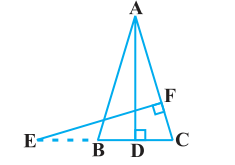# D is a point on the side BC of a triangle ABC such that ∠ADC = ∠BAC. Show that CA2 = CB.CD

Given that

D is a point on the side BC of a triangle ABC such that ∠ADC = ∠BAC.

To Prove

CA2 = CB.CDProof

∠ACD = ∠BCA (Common angles)

∴ ΔADC ~ ΔBAC (From AA similarity criterion)

We know that the corresponding sides of similar triangles are in proportion.

∴ CA/CB = CD/CA

Hence

⇒ CA2 = CB.CD.

Hence, proved.